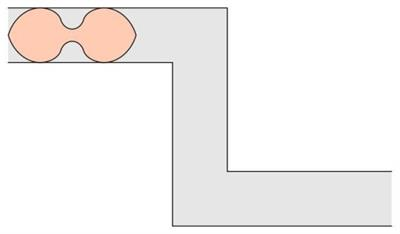Search - School / College / Institute

# New twist to the Sofa ProblemMost of us have struggled with the mathematical puzzle known as the "moving sofa problem." It poses a deceptively simple question: What is the largest sofa that can pivot around an L-shaped hallway corner?

For years, this mathematical problem has puzzled mathematicians and furniture movers. Mathematician Dan Romik has extended this problem to a hallway with two turns. He has shown that an infinity shaped sofa is the largest so far found that can move down such a hallway.

The largest area that will fit around a corner is called the "sofa constant". It is measured in units where one unit corresponds to the width of the hallway. To solve the problem, Romik has used the 3-D print technology. 3-D prints of both, the hallway and a sofa have been redesigned to get a shape that can easily crossover an L- shaped hallway.

“It is amazing how 3D print technology can be used to derive solutions to one of the biggest mathematical problems,” says Romik.

Image: https://phys.org/news/2017-03-sofa-problem-stumped-mathematicians-furniture.html### Future Bright Program

Enhance Your Skills With Our ExpertsInteractive School Platform